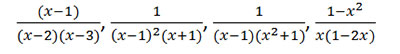#### Syllabus for test on Mathematics

Applicants from Commerce background opting for BCA programmes should qualify in-house Mathematics test to proceed with final admission. This test is not applicable for students who have studied Mathematics subject in class 12. The date of the Mathematics test will be informed to candidates shortlisted after the counselling / interview.

Please Note, Only those candidates who have confirmed their admission by remitting the tuition fee for first year and submitting the documents will be allowed to take the Mathematics test.

Prerequisites for Mathematics (for BCA course)
Matrices & Determinants: Addition, subtraction and multiplication of matrices;
Evaluation of determinants, finding inverse of a 3*3 matrix using the formulaQuadratic Equations: Completing squares of a quadratic polynomial, solution by factorization and by using formula.

Partial Fractions: Resolving rational algebraic fractions into partial fractions. Eg:

Analytic Geometry in 2D:Distance formula, section formula

Vectors: Addition of vectors, dot product

Trigonometry: Values of trigonometric functions of standard angles and allied angles, trigonometric identities (formulae attached)

Differential Calculus: Formulae of standard derivatives (attached), problems using product rule, quotient rule, chain rule.

Integral Calculus: Formulae of standard integrals (attached), integration by substitution method, evaluation of definite integrals.

Book for Reference: A Text Book of Mathematics, G.K Ranganath, Himalaya Publishing House. (1st and 2nd Year PUC)